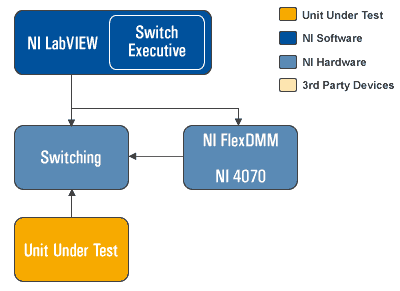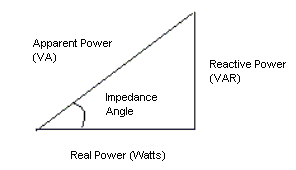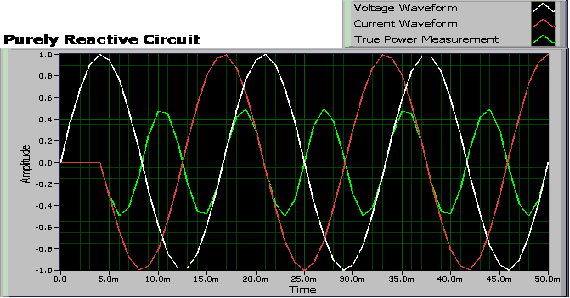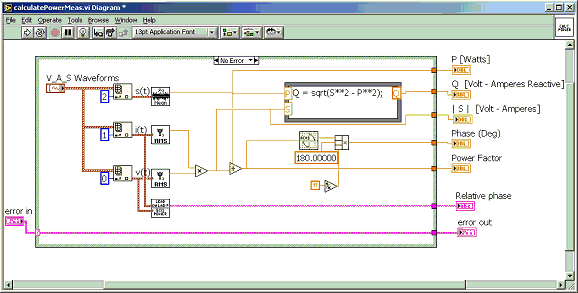# Measure Reactive Power with a Digital Multimeter

Publish Date: Aug 07, 2009 | 20 Ratings | 3.30 out of 5 | Print | Submit your review• NI LabVIEW
• NI 4070 FlexDMM

A power measurement application requires one measurement device to capture the voltage across the terminals of a load, and the second device to capture the current going through the load. However, the actual power calculation depends on the resistive and reactive components (capacitors and/or inductors) in the circuit. The power dissipation in a purely resistive circuit is always a function of the voltage drop and current draw through the circuit.

Reactive circuits appear to function like resistive circuits because they produce voltage drops and draw current. However, reactive circuits actually store or return power. The reactive components cause a phase shift (up to 90 degrees) between the voltage and current waveforms which reduces the overlap between the two curves and effectively delivers less power to the loads. This phenomenon is represented by three different power measurements: reactive power, apparent power, and real power. These three power measurements have a phase relationship that can be visualized in the power triangle, shown below.Figure 1: Power Triangle

Reactive Power
Reactive power is the measure of a circuit's reactance (X). A purely reactive circuit dissipates zero real power because the power is absorbed by the circuit and returned to the AC source. This circuit results in 90 degrees phase shift between the voltage and current waveforms, as shown in Figure 2. A circuit with resistive and reactive components will both dissipate power and return power to the AC source. The current and voltage waveforms will have a phase shift between 0 and 90 degrees.Figure 2: True Power in a Purely Reactive Circuit

Reactive power is represented with Q and has a unit measure of Volt-Amps-Reactive (VAR). You use the following formula to calculate reactive power:

Reactive Power (Q) = SQRT[( Apparent power)2 - (Real Power)2] VAR

To accurately measure reactive power, you need the following capabilities:
• Voltage and current waveform acquisition capability
• Simultaneous acquisition of both measurement waveforms
• Both measurement devices must acquire simultaneously
• Analysis functions

Traditionally, this measurement functionality was only found in specialized power meters because a traditional digital multimeter has asynchronous clocks and doesn't provide waveform acquisition capabilities. However, using the modern digital multimeter architecture of the NI PXI-4070 FlexDMM, which boasts digitizer capability, along with NI LabVIEW analysis functions, you can build a true power measurement system, as shown in Figure 3. The system requires two PXI-4070 FlexDMMs: one configured to measure voltage and the other to measure current. Using the NI-DMM instrument driver, you can configure two FlexDMMs to trigger off the same PXI trigger line. The block diagram below is an example of how you would make these measurements in LabVIEW.Figure 3: LabVIEW Analysis VIs Used to Calculate Reactive Power